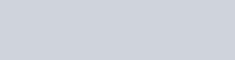Evaluate the following integrals:
Question:

Evaluate the following integrals:

$\int \frac{\sec x \operatorname{cosec} x}{\log (\tan x)} d x$

Solution: